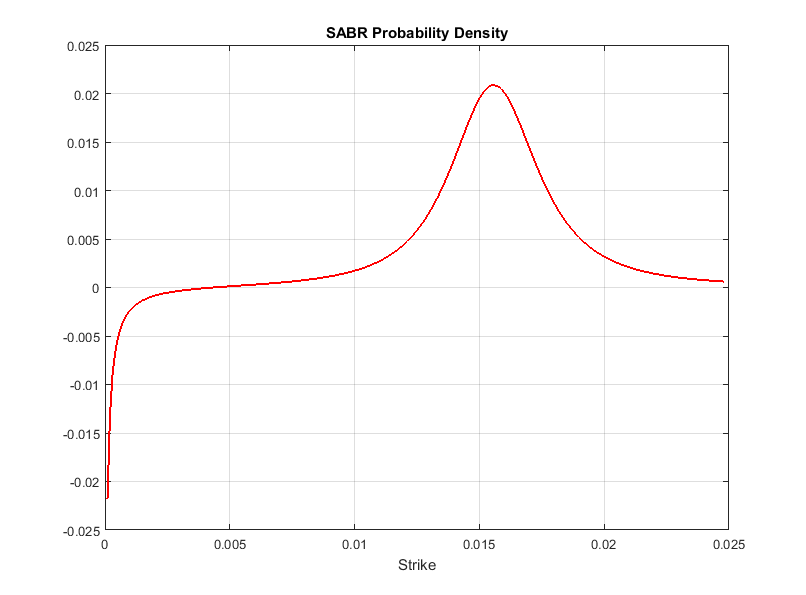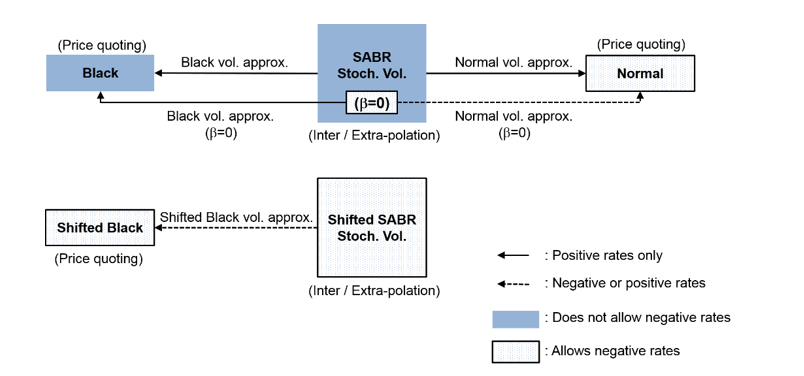Main Content

## Work with Negative Interest Rates Using Objects

### Interest-Rate Modeling Options for Negative Rates

Financial Instruments Toolbox™ computes prices for a `Cap`, `Floor`, or `Swaption` instrument when modeling for negative interest-rates using a Normal volatility model, shifted Black model, or shifted SABR model:

• Normal volatility model (Bachelier model) for interest-rate options to handle negative rates with the following:

• Shifted Black model and the shifted SABR model for interest-rate options to handle negative rates with the following:

• `Black` model object (Shifted Black model, specified by the `'Shift'` name-value argument set to a positive value.)

• `Black` pricer object (Shifted Black model, specified by the `'Shift'` name-value argument set to a positive value.)

• `SABR` model object (Shifted SABR model, specified by the `'VolatilityType'` name-value argument set to `"Black"` and the `'Shift'` name-value argument set to a positive value.)

• `SABR` pricer object (Shifted SABR model, specified by the `'VolatilityType'` name-value argument set to `"Black"` and the `'Shift'` name-value argument set to a positive value.)

### Modeling Negative Rates

The original authors of the SABR model provided a closed form approximation of the implied Black volatility in terms of the SABR model parameters (known as “Hagan’s formula”), so that the option price could be computed by inserting the computed SABR Black volatility into the Black formula:

`$Call\left(K,T\right)=Blac{k}_{call}\left(F,K,r,T,{\sigma }_{Black}\left(\alpha ,\beta ,\rho ,\nu ,F,K,T\right)\right)$`

However, these methods started to break down with the introduction of negative interest rates, due to the assumption of the Black model that the underlying rates are lognormally distributed (and therefore cannot be negative).

In addition, even when the underlying rate is positive, the closed form approximation of the SABR implied Black volatility (Hagan et al., 2002) is known to become increasingly inaccurate as the strike approaches zero. Even without crossing the zero strike boundary, the implied probability density of the underlying rate at option expiry can become negative at low positive strikes, although probability densities clearly should not be negative:Options with negative strikes cannot be represented by Black volatilities. To work around this problem, the market started to quote the cap, floor, and swaption prices also in terms of either Normal volatilities or Shifted Black volatilities. Instead of the Black model, both types of volatilities come from alternative models that allow negative rates.

#### Normal Model

The Normal volatilities are associated with the Normal model (also known as the Bachelier model):where the underlying rates are assumed to be normally distributed. Unlike in a lognormal model (where rates have a lower bound), the rates in the Normal model can be both infinitely positive and infinitely negative.

#### Shifted Black

The Shifted Black volatilities are associated with the Shifted Black model (also known as “Displaced Diffusion” or “Shifted Lognormal” model):The Shifted Black model is essentially the same as the Black model, except that it models the movements of (F + Shift) as the underlying asset, instead of F (where F is the forward swap rate in the case of swaptions, and the forward rate in the case of caplets and floorlets). So, the Shifted Black model allows negative rates, with a fixed negative lower bound defined by the amount of shift, that is, the zero lower bound of the Black model has been shifted.

#### Normal SABR and Shifted SABR

The introduction of negative interest rates also called for an update in the method for interpolating the volatilities quoted in the market. The following shows the connections between the volatilities and the SABR models:As shown, the Black and Normal volatility approximations allow you to use the SABR model with the Black and Normal model option pricing formulas. However, although the Normal model itself allows negative rates and the SABR model has an implied Normal volatility approximation, the underlying dynamics of the SABR model do not allow negative rates, unless β = 0. When the β (Beta) parameter of the `SABR` model is set to zero, the model is a Normal SABR model, which allows computing the implied Normal volatilities for negative rates.

In the Shifted SABR model, the Shifted Black volatility approximation can be used to allow negative rates with a fixed negative lower bound defined by the amount of shift. This is achieved by setting the `'Shift'` name-value argument of the `SABR` model to a positive value.

#### Implied Volatilities and SABR

You can compute the implied volatilities in terms of the SABR model parameters, for either β = 0 (Normal SABR), or any other value of β allowed by the SABR model (0 ≤ β ≤ 1) using the `volatilities` function for the `SABR` analytic pricer.

The following three types of implied volatilities are supported by the `SABR` analytic pricer, and the type of implied volatilities computed by the `volatilities` function depends on the parameters of the `SABR` model when using the `SABR` analytic pricer:

• Implied Black volatilities — The `SABR` model `'VolatilityType'` name-value argument is set to `"Black"` and the `'Shift'` name-value argument is set to zero. Negative rates are not allowed.

• Implied Sifted Black volatilities — The `SABR` model `'VolatilityType'` name-value argument is set to `"Black"` and the `'Shift'` name-value argument is set to a positive value. Negative rates are allowed with lower bound defined by the amount of shift.

• Implied Normal (Bachelier) volatilities — The `SABR` model `'VolatilityType'` name-value argument is set to `"Black"` and the `'Shift'` name-value argument is set to zero. Negative rates are allowed when the `Beta` input argument is set to zero.

Download ebook